# Real Contact Area

## Finite Element Analysis on Real Contact Area of 3D Rough Surface

This project applied finite element method to simulate and observe the activities of the interface of rough surface during the approcahing of 3D rough surface.

### The Modeling of Rough Surface Solid

#### The Construction of Rough Surface Models

• We generated 3D rough surface models based on experimental data, Gaussian rough surface model and fractal rough surface model.
• The real rough surface model was generated according to the data of a previous experiment conducted by Dr. Baojiang Song.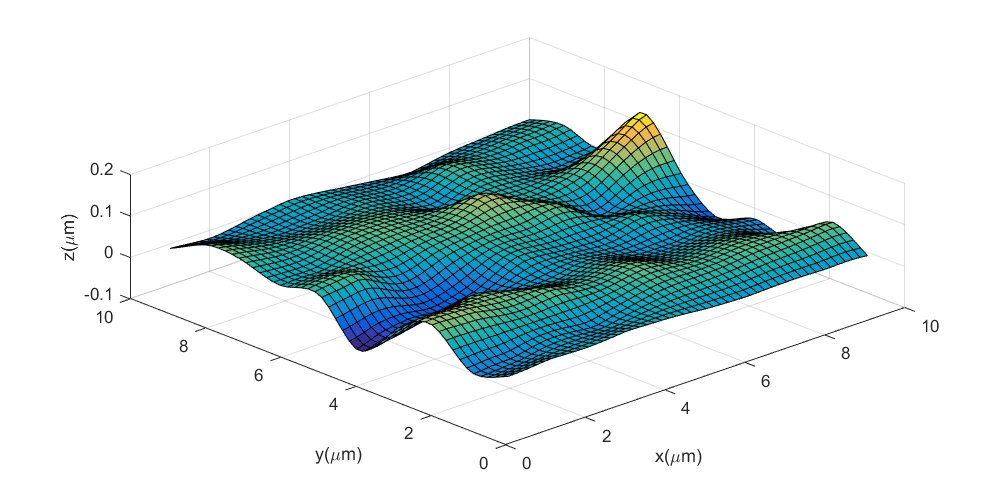• By employing the method described in , a Gaussian Rough Surface with a specified autocorrelation function can be generated by computer analog technology. The detailed method is discussed hereinbelow:where $\tau_x=1,2,\cdots,N$; $\tau_y=1,2,\cdots,N$; $n=N/2$, $m=M/2$, $h(\tau_x,\tau_y)$ is the impulse response function.
• The fractal surface model can be generated with the function in :

#### The Construction of Rough Surface Solid

• First, the 3D spot coordinated data obtained in the previous section with Matlab was further treated in an open source software, Meshlab, which can be used to edit and repair models, with functions of simplification, subdivision, sampling, cleaning and reconstruction.
• Then the generated 3D rough surface entity models could be import into 3D modeling software. As the surface models are too complex to stretch directly, they are projected at a certain distance from their equivalent plane.
• Finally, the 3D solid models can be obtained by stretching the projection to the original surface.
• To make the result more convincible, we scaled the solid models to keep their rough peak density and average height at the same order of magnitude.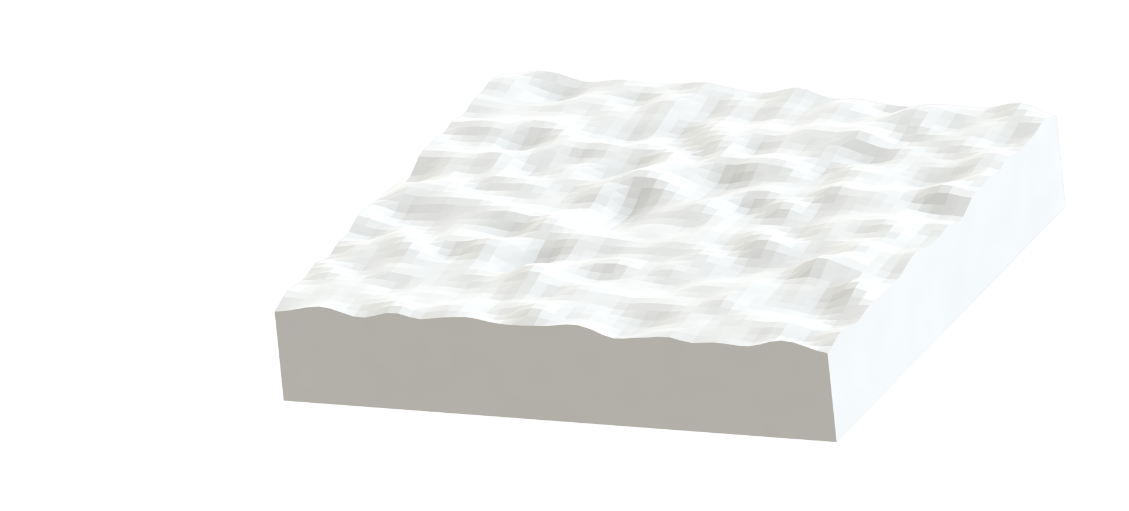The general process is described as below: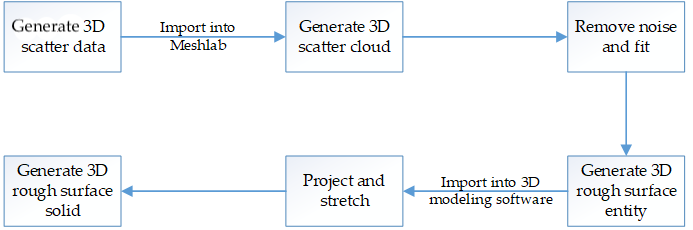### Finite Element Analysis

Here we use the software of ANSYS to implement the finite element analysis (FEA). This FEA software is developed by American company ANSYS and integrates structural, electric field, magnetic field, fluid and sound field analysis. We use ANSYS Workbench to study finite element analysis of the performance of the 3D rough surface during contact. To simulate an experimental condition of continuously loading, we chose transient analysis module to analysis the contact problem and the procedure is shown below: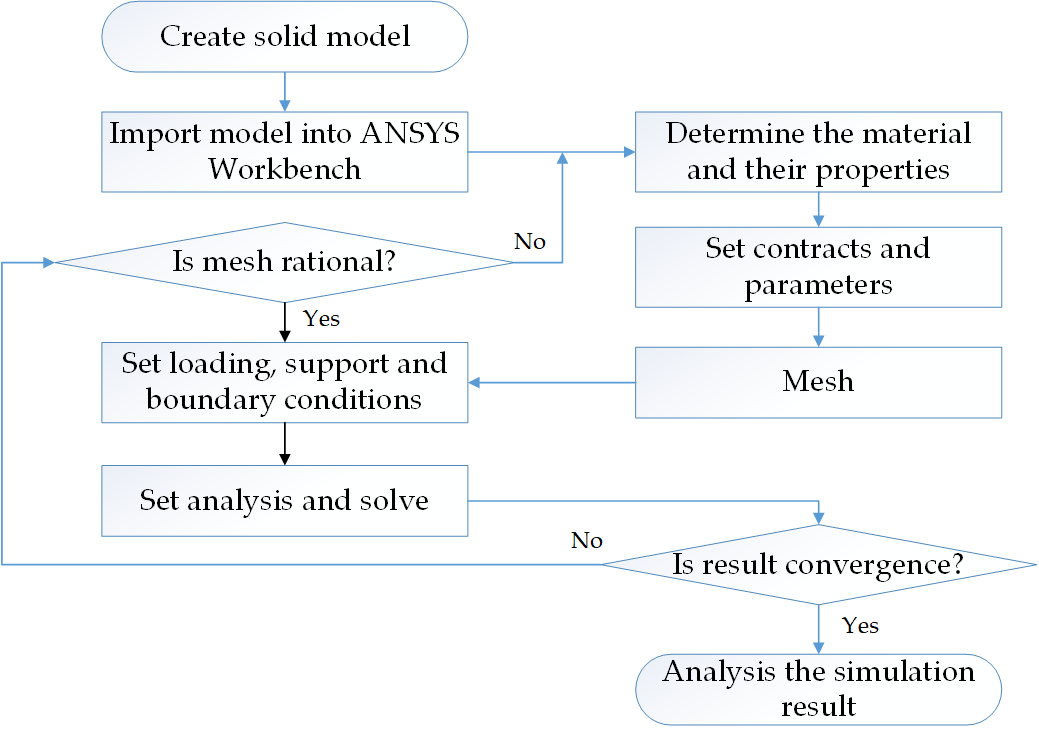• We analyzed obtained the strain field graph. When the strain in a pixel is greater than a threshold value, the cell it represents is in contact.
• The result of Gaussian surface and fractal surface is displayed. By comparing the result with that of real surface and a previous experiment, we can draw the conclusion that the fractal surface can better describe the rough surface in terms of contact characteristic.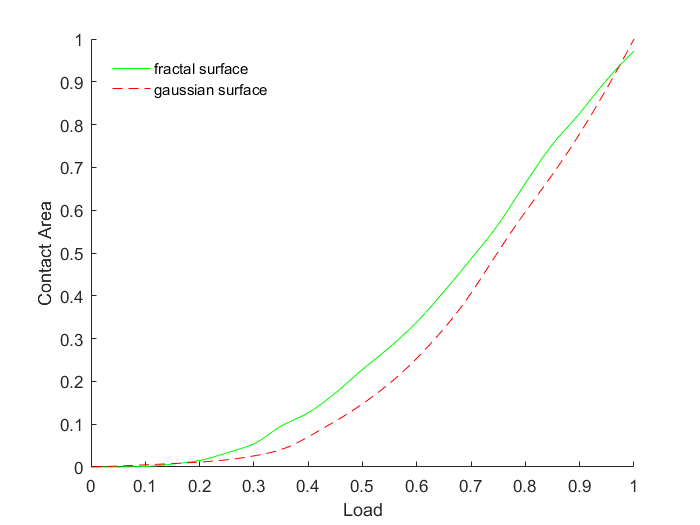### Acknowledgement

• All the work in this project was accomplished by me, under the instruction of Prof. Shaoze Yan, Dr. Baojiang Song and Zhijun Luo, Tsinghua University.

1. 1.C. Hui, H. Yuanzhong, W. Hui, W. Wenzhong, Computer simulation233of rough surfaces, LUBRICATION ENGINEERING-HUANGPU- 10234(2006) 52.
2. 2.Y. D. Liu, L. Liu, W-m fractals interpolation for anisotropic surface,308Science Technology Engineering (2011).
Share